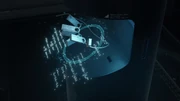FANDOM

115 Pages

 This article is a stub.It is too short to provide more than rudimentary information about a subject.You can help Death Stranding Wiki by expanding it.Sam's Q-pid is used to establish strands in the Chiral Network

A Q-pid is a necklace bearing a series of tag-engraved physics equations, containing all of the necessary security and operations protocols to integrate a terminal location into the Chiral Network. All established strand points are accessible to those bearing the required equations.

Sam is given a Q-pid upon undertaking his expedition across America.

List of equationsEdit

Inscribed equations are processed in the following order, and include:

1. $\partial_t q = \underline{\underline{D}} \,\nabla^2 q + R(q)$
Reaction-diffusion equation, represented in the general form.
2. $i \gamma^\mu \partial_\mu \psi(x) - m \psi(x) = 0$
Dirac equation, a part of quantum mechanics relating to Einstein's special relativity. It predicted the existence of antimatter.
3. $\phi = (\frac{\phi_1}{\phi_2})$
Higgs field equation, in a simplified form.
4. $G_\mu + \Lambda g_{\mu\nu} = \kappa T_{\mu\nu}$
Einstein field equation, which describes gravitation, and notably uses Einstein's gravitational constant $\kappa$.
5. Quantum entanglement state.
6. $r_g = \frac{2 G M}{c^2}$
Schwartzschild radius, the radius to which an object would have to be compressed (i.e., increasing its density without losing any of its mass) in order to turn it into a black hole.

After the above equations are processed, the Schrödinger equation and an equation related to Lagrangian density, along with others, are then additionally loaded and displayed.Edit

Community content is available under CC-BY-SA unless otherwise noted.